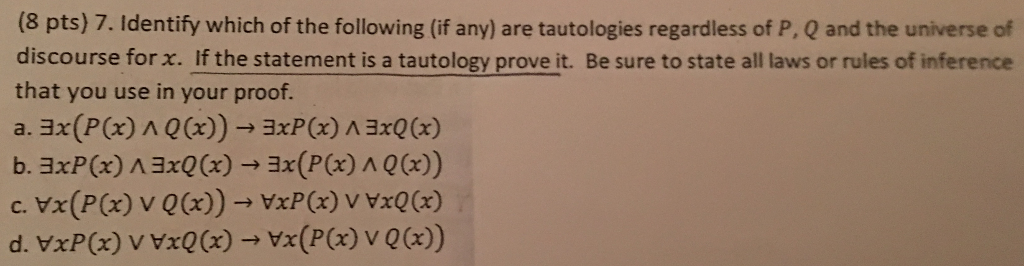# Answered! (8 pts) 7. Identify which of the following (if any) are tautologies regardless of P, Q and the universe of discourse for x….Identify which of the following are tautologies….

Don't use plagiarized sources. Get Your Custom Essay on
Answered! (8 pts) 7. Identify which of the following (if any) are tautologies regardless of P, Q and the universe of discourse for x….
GET AN ESSAY WRITTEN FOR YOU FROM AS LOW AS \$13/PAGE

Apparently a. and d. are tautologies, but I cannot understand why so I do not know how to prove either.

(8 pts) 7. Identify which of the following (if any) are tautologies regardless of P, Q and the universe of discourse for x. If the statement is a tautology prove it. Be sure to state all laws or rules of inference that you use in your proof. b. axPOx) A3xQ(x) 3x(P(x), A, Q(x))

For all iplication rules we need to test for only one case,when left hand side of the implication is 1 and right hand side is 0 that will result false.

a)
LHS: this is true only when there exist x (let it be x1) for which both P(x) and Q(x) holds true (i.e. P(x1) = true, and Q(x1) = true)

RHS: If LHS is true, we cannot make LHS false (since we have atleast one x, for which both P() and Q() are true. So obhiously there exist a x for which P() is true and there exist a x for which Q() is true)

So this is Tautology

b)
LHS: This is true when there exist a x (let it be x1) for which P(x) is true and there exist a x,may be different, (let it be x2) for which Q(x) is true.

RHS: If LHS is true, we cannot be sure RHS is true, because RHS demands there exist x (let it be x1) for which both P(x) and Q(x) holds true (i.e. P(x1) = true, and Q(x1) = true)

so this is not Tautology

c)
LHS: is True when for all x either P(x) is true or Q(x) is true.

RHS: If LHS is true we cannot comment on RHS, since RHS demands for all x P(x) is true or for all x Q(x) is true. (difference is in LHS it states that we can have x1 such that P(x1) is false, but Q(x1) should be true or vice-versa. But RHS says either P(x) is true for all X or Q(x) is true for all X)
so this is not Tautology

d)
LHS:This is true if for all x P(x) is true or for all x Q(x) is true.

RHS:True value of LHS confirms RHS is True because RHS demands for all x either P(x) is true or Q(x) is true.

so this is Tautology

I hope you like the explanation provided. If you have and doubt please feel free to comment below. I shall be glad yo help you